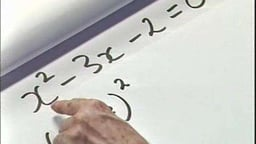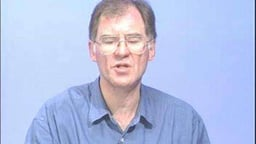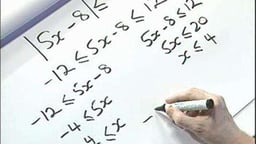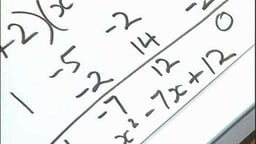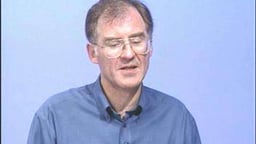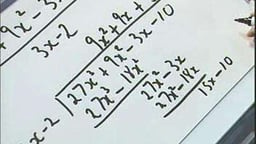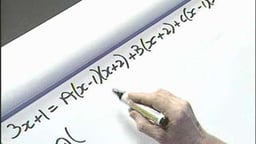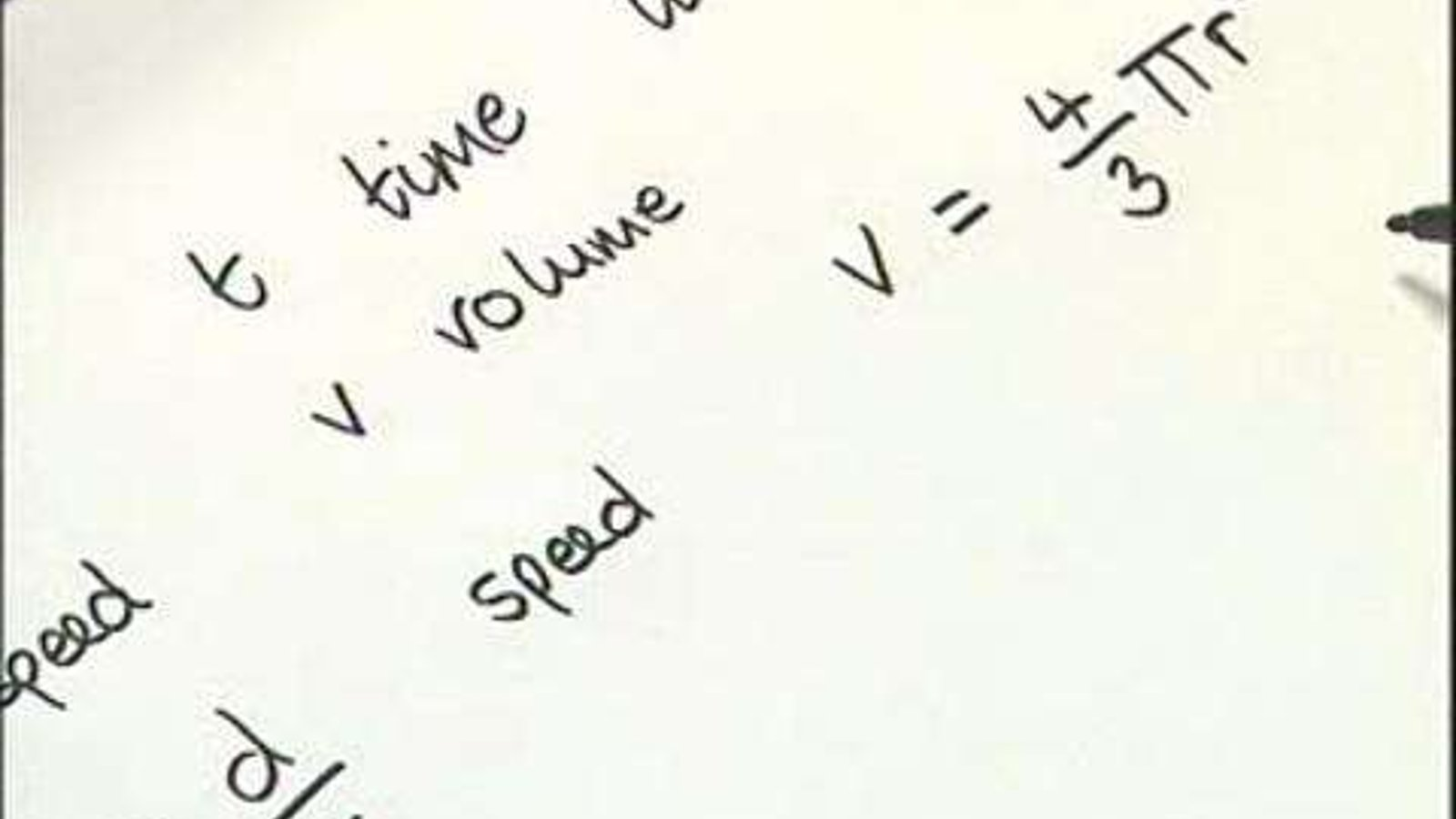Algebra

2007The 18 videos in this collection focus on all aspects of Algebra, from logarithms to quadratic equations and partial fractions.

Supplier
FENC

In This Series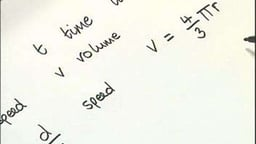Mathematical Language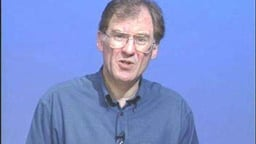Powers or Indices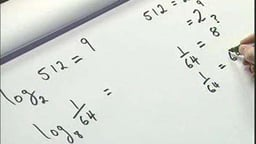Logarithms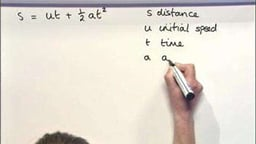Substitution and Formulae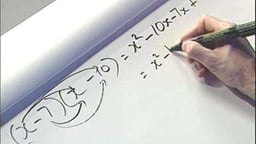Expanding and Removing Brackets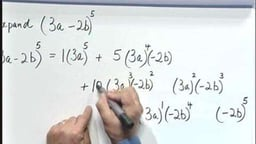Pascal's Triangle and the Binomial Theorem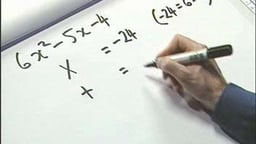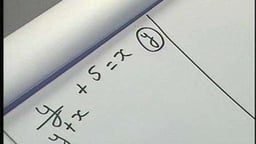Transposition of Formulae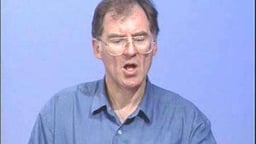Linear Equations in One Variable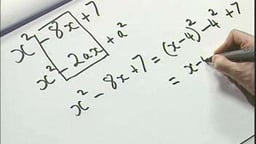Completing the SquareCompleting the Square (max, min)